Arbitrage Identification Strategies

March 29th @ Slash Hackathon 2019Questions? Contact: roman@celo.org

# Representations of a Market¶

Assume that there is a market with $N$ currencies. Also assume for simplicity that every possible pair can be traded (not a necessary assumption for the depicted representations). The exchange rates can be displayed by a source-to-target exchange rate matrix. Let us generate and analyze an exemplary such matrix and translate it into a graph representation.

In :
# set things up and create button

In :
# set some parameters, generate exchange rate matrix and the corresponding graph
N = 6 # number of currencies
max_spread_pct = 0.05 # maximum bid-ask spread in pct of bid, 0.05 for 5%


## Exchange Rate Matrix Representation¶

The exchange rate matrix takes the current best bids and best asks in the market and generates a matrix of multipliers that shows how many units of the target currency one would receive for a unit of the source currency.

In :
# generate and display random exchange rate matrix

target
0 1 2 3 4 5
source 0 1.00 0.58 0.63 0.27 0.17 0.20
1 1.68 1.00 0.39 0.74 0.47 0.79
2 1.57 2.48 1.00 0.04 0.15 0.11
3 3.64 1.35 22.94 1.00 0.22 0.23
4 5.84 2.02 6.84 4.40 1.00 0.49
5 4.76 1.21 8.59 4.41 1.97 1.00

## Directed Graph Representation¶

The above exchange rate matrix can be translated into a directed graph with nodes representing the currencies and the edge weights set to the exchange rate matrix multipliers.

In :
# display corresponding directed graph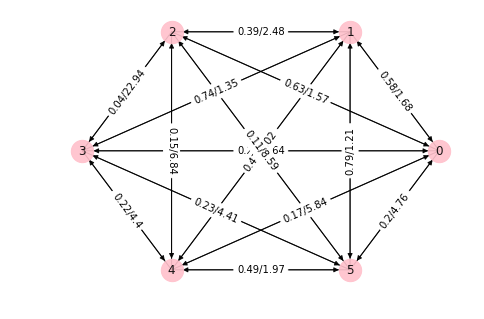An arbitrage is a cylce for which the cumulative product of the edge weights in an exchange rate graph is bigger than one.

## Log-Transformed Representations¶

There are many well known graph algorithms that deal with cycle lengths - the cumulative sum of the edge weights. Fortunately, one can easily translate a cumulative product optimization problem into a convex cumulative sum optimzation problem by taking logs. To see this, note that for $a>0<b$ \begin{align} ln\left(a\times b \right) = ln\left(a\right) + ln\left(b\right) \end{align}

while noting that \begin{align} ln\left(x\right) \quad \text{is a positive and monotonic transformation.} \end{align}

If we multiply everything with -1, convex maximization becomes convex minimization... Let us transform the above exchange rate matrix and the corresponding graph by taking negative logs.

In :
# display log-transformed exchange rate matrix and corresponding graph

Taking negative logs...

target
0 1 2 3 4 5
source 0 -ln(1.0) -ln(0.58) -ln(0.63) -ln(0.27) -ln(0.17) -ln(0.2)
1 -ln(1.68) -ln(1.0) -ln(0.39) -ln(0.74) -ln(0.47) -ln(0.79)
2 -ln(1.57) -ln(2.48) -ln(1.0) -ln(0.04) -ln(0.15) -ln(0.11)
3 -ln(3.64) -ln(1.35) -ln(22.94) -ln(1.0) -ln(0.22) -ln(0.23)
4 -ln(5.84) -ln(2.02) -ln(6.84) -ln(4.4) -ln(1.0) -ln(0.49)
5 -ln(4.76) -ln(1.21) -ln(8.59) -ln(4.41) -ln(1.97) -ln(1.0)

...results in:

target
0 1 2 3 4 5
source 0 0.000000 0.544727 0.462035 1.309333 1.771957 1.609438
1 -0.518794 0.000000 0.941609 0.301105 0.755023 0.235722
2 -0.451076 -0.908259 0.000000 3.218876 1.897120 2.207275
3 -1.291984 -0.300105 -3.132882 0.000000 1.514128 1.469676
4 -1.764731 -0.703098 -1.922788 -1.481605 0.000000 0.713350
5 -1.560248 -0.190620 -2.150599 -1.483875 -0.678034 0.000000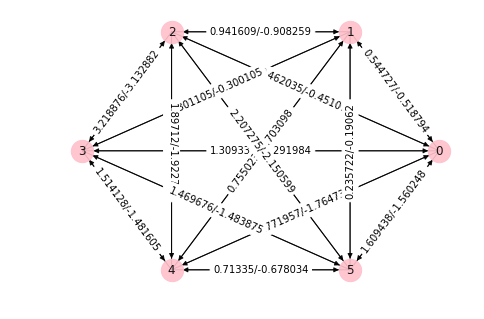Every cycle of negative length in the above graph with negative-log-transformed weights is an arbitrage cylce.

# Identifying Arbitrage Cycles¶

## Finding an Arbitrage Cycle with Bellman-Ford¶

One can check if an arbitrage cylce exists and if so, identify one such a cylce in the log-transformed graph representation using a slighlty adjusted Bellman-Ford algorithm which has a complexity of $\mathcal{O}(|V||E|)$ where $|V|$ denotes the number of vertices and $|E|$ denotes the number of edges. A neat visualization of how the Bellman-Ford algorithm works can be found here.

The Bellman-Ford algorithm itself only gives the shortest distance from a prespecified source node to all other nodes in the graph. It needs to be adjusted to:

• show if there is a negative cycle
• to output a negative cycle
• to always find a negative cycle (if there is one) even the graph is not connected
In :
# The Bellman-Ford function used in this notebook
def bf_negative_cycle(G):

# Remove nan edges
n = len(G.nodes()) + 1
edges = [edge for edge in G.edges().data() if ~np.isnan(edge['weight'])]

# Add a starting node and add edges with zero weight to all other nodes
start_node_edges = [(n-1, i, {'weight': 0}) for i in range(n-1)]
edges = edges + start_node_edges

# Initialize node distances and predecessors
d = np.ones(n) * np.inf
d[n - 1] = 0  # Starting node has zero distance
p = np.ones(n) * -1

# Relax n times
for i in range(n):
x = -1
for e in edges:
if d[int(e)] + e['weight'] < d[int(e)]:
d[int(e)] = d[int(e)] + e['weight']
p[int(e)] = int(e)
x = int(e)
if x == -1:  # If no relaxation possible, no negative cycle
return None

# Identify negative cycle
for i in range(n):
x = p[int(x)]
cycle = []
v = x
while True:
cycle.append(int(v))
if v == x and len(cycle) > 1:
break
v = p[int(v)]
return list(reversed(cycle))

In :
# Check if arbitrage cycle exists using Bellman-Ford. If so, output an arbitrage cycle

At least one arbitrage cycle exists! One such cycle is [2, 1, 5, 3, 2] with cycle length -5.2893 which implies and arbitrage multiplier of 198.2033.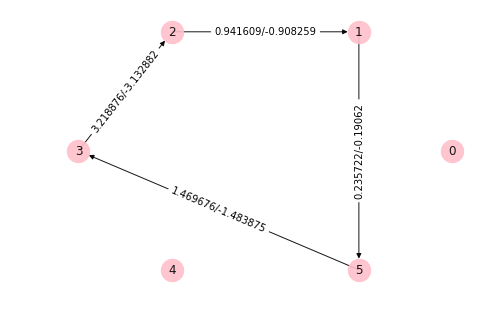## Finding an "Optimal" Set of Cycles¶

Identifying the cycle with an arbitrary number of vertices with the largest arbitrage multiplier is NP-hard in general. Finding this cycle with many tradable pairs thus quickly becomes computationally infeasible and one has to resort to approximate/heuristic algorithms.

Observe that finding the cycle with the largest multiplier (i.e. the cycle with the most negative cycle length in the graph with negative-log-transformed weights) of all cycles that visit each vertex exactly once is equivalent to the (asymmetric) Travelling Salesman Problem - a well known NP-hard problem. The Travelling Salesman Problem (TSP) with $n+1$ cities can be formulated as an integer linear program for a distance matrix $C$ as follows \begin{align} \min &\sum_{i=0}^n \sum_{j\ne i,j=0}^nc_{ij}x_{ij} && \\ & 0 \le x_{ij} \le 1 && i,j=0, \cdots, n \\ & u_{i} \in \mathbf{Z} && i=0, \cdots, n \\ & \sum_{i=0,i\ne j}^n x_{ij} = 1 && j=0, \cdots, n \\ & \sum_{j=0,j\ne i}^n x_{ij} = 1 && i=0, \cdots, n \\ &u_i-u_j +nx_{ij} \le n-1 && 1 \le i \ne j \le n \end{align}

The TSP is only NP-hard because we are asking for a single roundtrip solution that covers all cities / currencies (the last two sets of constraints in the above formulation). But subtours are fine in the arbitrage finding task and even skipping some assets completely (a self-cylce) is not a problem!

If we remove the "no-subtours" constaints of the TSP and also account for the diagonal of $X$ in the objective function, we arrive at the linear programming relaxation of the assignment problem \begin{align} \min &\sum_{i=0}^n \sum_{j=0}^nc_{ij}x_{ij} && \\ & 0 \le x_{ij} \le 1 && i,j=0, \cdots, n \\ & \sum_{i=0}^n x_{ij} = 1 && j=0, \cdots, n \\ & \sum_{j=0}^n x_{ij} = 1 && i=0, \cdots, n \\ \end{align} This linear program always has an integer solution (due to the totally unimodular linear constraint matrix) and thus solves the assignment problem. You can use a very specialized solver for the assignment problem (this notebook uses this one - extremely fast and exact solutions!) or just solve the linear program above - CVXPY makes this very easy.

In :
# Alternative: Solve Assignment Problem with CVXPY
X = Variable((len(C_ln),len(C_ln)))
iota = np.ones((len(C_ln),1))

constraints = [X <= 1,
X >= 0,
X*iota == 1,
np.transpose(iota)*X == 1]

# Form objective.
obj = Minimize(sum(multiply(X, C_ln)))

# Form and solve problem.
prob = Problem(obj, constraints)
prob.solve()  # Returns the optimal value.
print ("status:", prob.status)
print ("optimal value", prob.value)
X = pd.DataFrame(X.value)
display(X.round(3))

status: optimal
optimal value -5.965679824113291

0 1 2 3 4 5
0 1.0 -0.0 0.0 -0.0 0.0 0.0
1 -0.0 -0.0 -0.0 0.0 0.0 1.0
2 0.0 1.0 -0.0 -0.0 -0.0 -0.0
3 -0.0 0.0 1.0 -0.0 0.0 0.0
4 0.0 0.0 -0.0 1.0 -0.0 -0.0
5 0.0 0.0 0.0 0.0 1.0 -0.0
In :
# Find best set of arbitrage cycles by solving the assignment problem

 Optimal cycle set multiplier (assumes one has enough quantities in every sub-cycle): 389.5750080640003.
Multiplier is garuanteed to be at least as large as the multiplier found by Bellman-Ford (198.2033 in this example).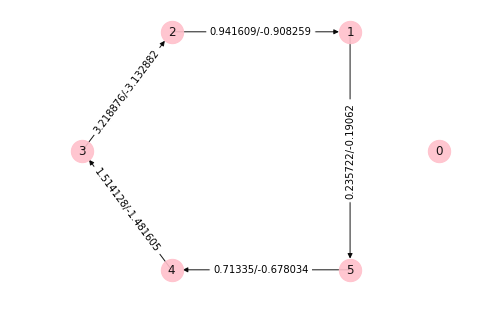## Beyond Arbitrage Cycles - Maximize wrt. Quantities (EXPERIMENTAL!)¶

Assume that we start out with one quantity in each currency. Let us find the set of trades that maximize the quantity in currency $k$ without changing the other quantities. For two matrices $A$ and $B$ of the same dimensions, let $A\circ B$ denote the element-wise product (also denoted Hadamard product)).

Let $C$ denote the exchange rate matrix (not log-transformed) and $Q$ a matrix of the same size as $C$ where the entry $Q_{i,j}$ denotes the number of $i$ coins that flow from currency $i$ to currency $j$. Let $\mathbb{1}$ denote a vector of ones of size $N\times 1$, then vector of outflows from the respective currency inventories is

\begin{align} \text{outflows} = Q \mathbb{1}. \end{align}

The vector of inflows (also in currency $i$ units) is

\begin{align} \text{inflows} = (Q \circ C)' \mathbb{1} \end{align}

and hence the delta inventory is

\begin{align} \Delta\! I = (Q \circ C)' \mathbb{1} - Q \mathbb{1}. \end{align}

Let $I_{init}$ denote the initial inventory the maximizre

\begin{align} \max_{Q} \Delta\! I_k && \\ & \Delta\! I_{\neq k} =0 && \text{| No change in inventory in other assets}\\ & \Delta\! I_k \leq 1000 && \text{| Problem would be unbounded in case of arb-cycle}\\ \\ \end{align}

The nice thing about this setup is that it allows to easily incorperate new constraints like the blocksize of the observed quotes. The drawback is that this is numerically extremely challenging!

In :
# Maximize inventory

target
0 1 2 3 4 5
source 0 1.00 0.58 0.63 0.27 0.17 0.20
1 1.68 1.00 0.39 0.74 0.47 0.79
2 1.57 2.48 1.00 0.04 0.15 0.11
3 3.64 1.35 22.94 1.00 0.22 0.23
4 5.84 2.02 6.84 4.40 1.00 0.49
5 4.76 1.21 8.59 4.41 1.97 1.00
inventory_start
currency
0 1.0
1 1.0
2 1.0
3 1.0
4 1.0
5 1.0
seconds it took: 0.017813920974731445
status:  optimal
optimal value:  999.998326792761

Quantities matrix Q:

target
0 1 2 3 4 5
source 0 0 0.55526 1.18114 1.05574 0.823924 0.642941
1 33.4973 0 1.31654 0.516985 13.0599 95.599
2 70.3751 47.2097 0 0.352497 12.2592 12.3467
3 82.1541 17.0369 5.91057 0 0.519886 0.254038
4 70.0033 0.260328 0.330315 17.5992 0 1.1418
5 27.2338 2.53042 0.400214 6.29415 41.1693 0
currency
0 1.0 9.999983e+02 1000.998327
1 1.0 8.605809e-09 1.000000
2 1.0 1.377347e-06 1.000001
3 1.0 7.083011e-08 1.000000
4 1.0 -1.618656e-07 1.000000
5 1.0 -1.211123e-07 1.000000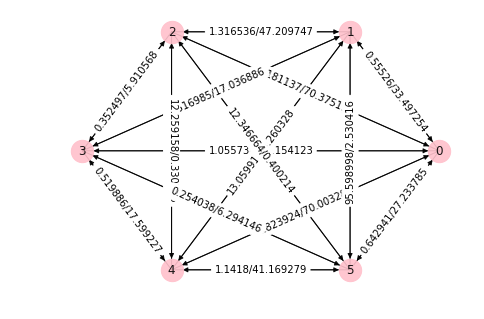# Live Arbitrage Identification¶

In this part, we are looking for live arbitrage opportunities on some centralized exchanges. The main tool we use for this is CCXT - an open source JavaScript / Python / PHP cryptocurrency trading library with support for more than 130 crypto exchanges.

The Binance fee structure can be found here.

In :
# set some parameters

In :
# set things up and create button

In :
# create real time exchange rate matrix

Updating all quotes (496 pairs overall) took 0.9450349807739258 seconds.

In :
# Check if arbitrage cycle exists using Bellman-Ford. If so, output an arbitrage cycle

Bellmann-Ford took 0.22376108169555664 seconds.
At least one arbitrage cycle exists! One such cycle is ['BTC', 'CDT', 'ETH', 'BTC'] with cycle length -0.000546627229625 which implies and arbitrage multiplier of 1.0005467766575147.

Node 0 1 2
Ticker BTC CDT ETH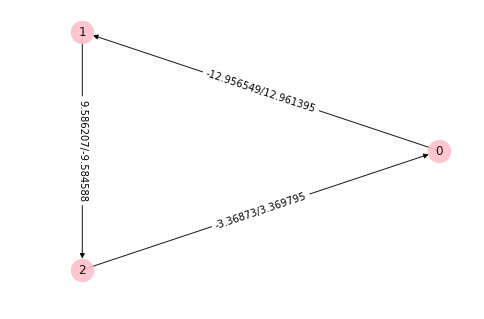In :
# Find best set of arbitrage cycles by solving the assignment problem

Solving assignment problem took 0.053289175033569336 seconds.

Assignment problem multiplier is 1.0006195840057635.

Node 0 1 2 3 4 5
Ticker ADA BTC CDT ETH NEO USDT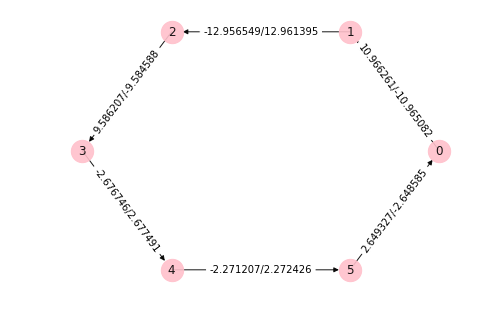# Practical Challenges of Arbitrage Trading¶

Aspects to consider:

• Speed, speed, speed!
• Timing issues
• Costs of inventory (opportunity cost, market risk, custody risk)
• Available liqudity
• Precision of quantities
• Error handling
• ...